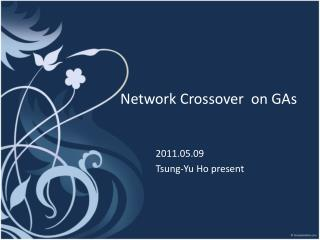DownloadDownload PresentationNetwork Crossover on GAs

# Network Crossover on GAs

Download Presentation## Network Crossover on GAs

- - - - - - - - - - - - - - - - - - - - - - - - - - - E N D - - - - - - - - - - - - - - - - - - - - - - - - - - -
##### Presentation Transcript

1. Network Crossover on GAs 2011.05.09 Tsung-Yu Ho present

2. Reference Paper • ‘’Network Crossover Performance on NK Landscapes and Deceptive Problems’’ • GECCO, 2010 • Mark Hauschild and Martin Pelikan

3. Motivation • GAs with uniform crossover • Break up too much linkages for tight linkage problems. • Need a problem-specified crossover operator • EDAs • Highly cost on model building • Specify the crossover operator according to model building

4. Goal • GAs with network crossover • Can solve hard problems than SGA • Reduce the model-building cost than EDAs • A general crossover-form without specification for problems.

5. Goal • GAs with network crossover • Can solve hard problems than SGA • Reduce the model-building cost than EDAs • A general crossover-form without specification for problems. Is it possible ?

6. Methodology Prior knowledge Clear Problem Information Matrix GAs Network Crossover The advantages as last slide mentions

7. Goal & Test Problems • Test Algorthms • GAs with network crossover • ... uniform crossover • ... 2-points crossover • hBOA (outperform ?) • Test problems • Trap-5 • NK Landscapes (overlapping problem)

8. Some Question about this paper • Page1,’’... This paper discusses a network crossover operator that works with a user-specified network graph to determine which bits are exchanged. In addition, due to not requiring a costly model building phase, it might be able to outpeform EDAs.’’ • Page2.’’...For those problems without an implict graph, it is possible to run an EDA on trial instances of the problems to learn a network structure. ’’

9. Network Crossover • Network crossover operator needs prior knowledge of structure information. • Graph for network crossover • Node (gene) , Edge(gen’s linkage) • Need an N x N matrix to record the relation • How to get this structure information? • Clear graph definition (edge between nodes) • Without an implicit graph problems • Run EDAs to get this graph.

10. Information Matrix • An N x N matrix G is given • For reducing information, only spedify the strongestconnection • Do not require the strength of each interaction • Only 0 or 1 in each entry • How to decide the strongest connection (G)? • Papers ‘’Using previous models to bias structural Learning in the hierarchical BOA’’, M. Hauschild, M. Pelikan, K. Sastry, and D. E. Goldberg, GECCO’2008.

11. Network Crossover Algorithm • Pick up n/2 mask for crossover in length n • Randomly choose one bit as start point. • From start point, use breadth first search to add the point

12. Network Crossover Algorithm

13. Network Crossover Algorithm 0 0 0 0 0 0 0 0 3 0 0 1 0 0 0 0 0 6 3 0 1 1 0 0 1 0 0 2 0 1 1 0 0 1 1 0 6 3 7 2

14. Network Crossover Algorithm

15. Test Problems • 5-bit trap problem • NK Landscapes problem • Unrestriced NK landscapes. • Nearest neighbor NK landscapes.

16. NK Landscapes • NK Landscapes problem • Unrestriced NK landscapes. • Nearest neighbor NK landscapes. : Number of neighbors per bit : the set of neighbors for bit

17. NK Landscapes • Unrestriced NK landscapes • Each position i, choose a random set of k neighbors with equality probability • A lookup table defining fi from the uniforn distribution over [0,1) • k = 5, in this test

18. NK Landscapes • Nearest neighbor NK landscapes • Bits are arange as a circle • k bits follow the i th bit K = 3 step = 1 step = k

19. Test Problems • 5-bit trap problem (k = 5) • NK Landscapes problem • Unrestriced NK landscapes (k = 5) • Nearest neighbor NK landscapes (k = 5, step = 1) • Nearest neighbor NK landscapes (k = 5, step = 5) • RTR & elitism to compare

20. Deterministic Hill Climber • Deterministic Hill Climber • Take a candidate solution • Performs one-bit changes on this solution that lead to maxmum improvement • Terminated until no improvement.

21. Result

22. Result

23. Result

24. Information Matrix • Papers ‘’Using previous models to bias structural Learning in the hierarchical BOA’’, M. Hauschild, M. Pelikan, K. Sastry, and D. E. Goldberg, GECCO’2008. • Generally speaking,if the structure information are not fully correct, it may lead to being unable sovle problem. • The paper give the strongest connection matrix, for network crossover.

25. Probability Coincidence Matrix • Probability Coincidence Matrix (PCM) • For each pair (i,j), the value of Pi,j means the proportion of of probabilistic models in which ith and jth string position are connected. • Incremete the count by 1, if each models has a connection on i and j. • P = 0.25 • 25% of the available probabilistic models contains a connection between i and j. • Use the threshold Pmin to divide value into 1 or 0.

26. Summary for PCM • EDAs provides a series of probabilistic models that hold a great deal of information about problems. • We can use these available probabilistic modelsas prior information to speed up model building on EDAs • The prior information can help network crossover.

27. Conclusion • GAs with network crossover to solve overlapping problem without model building like EDAs • A general form, that do not be modified for specified problems. • The most big problems is ‘’How to get the strongest information matrix’’.